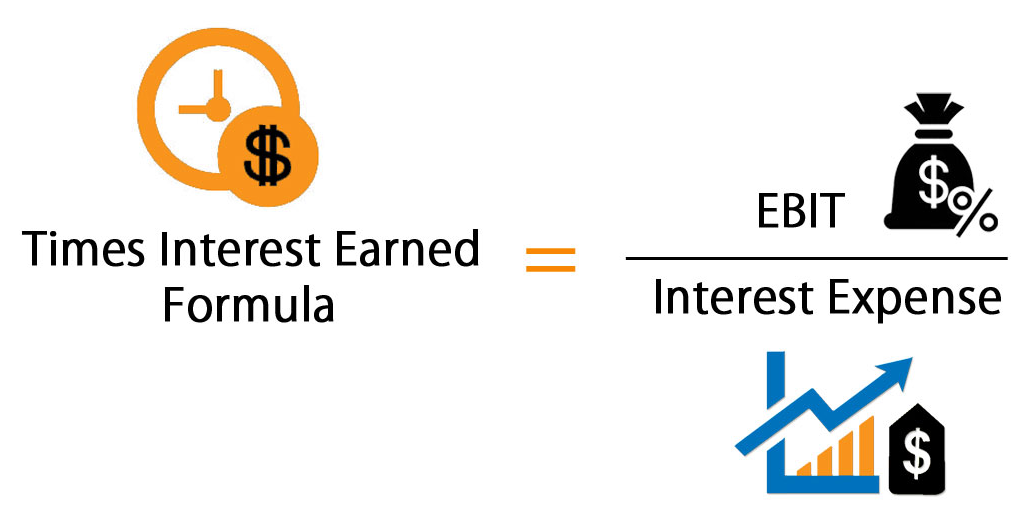# Times Interest Earned Ratio | Analysis | Formula

The times interest earned ratio is an indicator of a corporation’s ability to meet the interest payments on its debt. The times interest earned ratio is calculated as follows: the corporation’s income before interest expense and income tax expense divided by its interest expense.

The larger the times interest earned ratio, the more likely that the corporation can make its interest payments. The times interest earned ratio is also referred to as the interest coverage ratio.## Times Interest Earned Ratio Formula

Failing to meet these obligations could force a company into bankruptcy. TIE is also referred to as the interest coverage ratio. Generating cash flow to make principal and interest payments and avoiding bankruptcy depends on a company’s ability to produce earnings.

A company’s capitalization refers to the amount of money it has raised by issuing stock or debt and choices about capitalization impact the TIE ratio. Businesses consider the cost of capital for stock and debt and they use that cost to make decisions about capitalization.The times interest earned ratio is calculated by dividing income before interest and income taxes by the interest expense.

Both of these figures can be found on the income statement. Interest expense and income taxes are often reported separately from the normal operating expenses for solvency analysis purposes. This also makes it easier to find the earnings before interest and taxes or EBIT.

## Times-Interest-Earned Ratio Example

Assume that a corporation had the following amounts for the most recent year:

• Net income after tax of \$500,000
• Interest expense of \$200,000
• Income tax expense of \$300,000

Given these assumptions, the corporation’s income before interest and income tax expense was \$1,000,000 (net income of \$500,000 + interest expense of \$200,000 + income tax expense of \$300,000). Since the interest expense was \$200,000, the corporation’s times interest earned ratio was 5 (\$1,000,000 divided by \$200,000).

## Times Interest Earned Ratio Analysis

The times interest ratio is stated in numbers as opposed to a percentage. The ratio indicates how many times a company could pay the interest with its before tax income, so obviously the larger ratios are considered more favorable than smaller ratios.

In other words, a ratio of 4 means that a company makes enough income to pay for its total interest expense 4 times over. Said another way, this company’s income is 4 times higher than its interest expense for the year.

As you can see, creditors would favor a company with a much higher times interest ratio because it shows the company can afford to pay its interest payments when they come due. Higher ratios are less risky while lower ratios indicate credit risk.

## Times Interest Earned Ratio Calculator

Assume, for example, that XYZ Company has \$10 million in 4% debt outstanding and \$10 million in common stock and that the firm needs to raise more capital to purchase equipment. The cost of capital for issuing more debt is an annual interest rate of 6% and shareholders expect an annual dividend payment of 8%, plus appreciation in the stock price of XYZ.

The business decides to issue \$10 million in additional debt and the firm determines that the total annual interest expense is: (4% X \$10 million) + (6% X \$10 million), or \$1 million annually. The company’s EBIT calculation is \$3 million, which means that the TIE is 3, or three times the annual interest expense (interest payable).

## What Is Difference Between The Current Ratio And The Times Interest Earned Ratio?

### Current Ratio:

The current ratio is a liquidity ratio that measures a company’s ability to pay short-term obligations or those due within one year. It tells investors and analysts how a company can maximize the current assets on its balance sheet to satisfy its current debt and other payables.

### Times Earned Interest Ratio

Times interest earned (TIE) is a metric used to measure a company’s ability to meet its debt obligations. The formula is calculated by taking a company’s earnings before interest and taxes (EBIT) and dividing it by the total interest payable on bonds and other contractual debt. TIE indicates how many times a company can cover its interest charges on a pretax earnings basis.